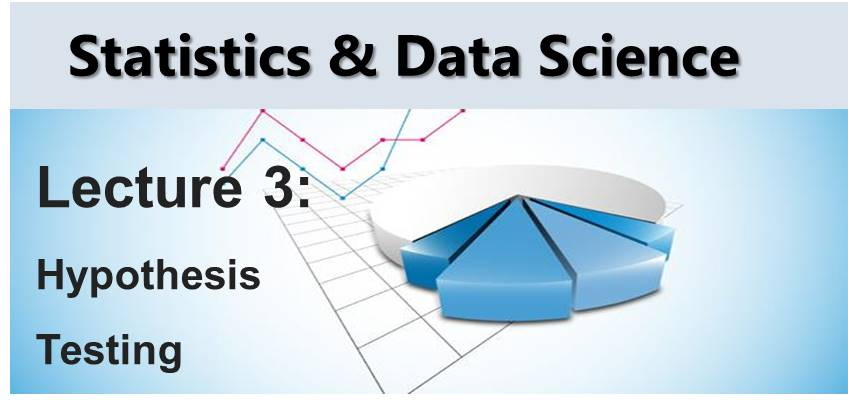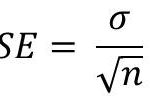# Statistics Tutorial 3 – Hypothesis TestingIn this tutorial we are going to cover Hypothesis Testing. To understand this topic better, we would break it down into the following sub-topics

Estimation is a statistical way of trying to deduce the value of an unknown parameter. For example, to estimate the mean of a population µ, we can take a sample from the population and calculate the mean. Then we can use the sample mean as an estimate of the population mean.

Point Estimate vs Interval Estimate

A point estimate is one single number that is represents the parameter you are trying to estimate.

Interval estimates is a range of values that represents the parameter you are trying to estimate. Hence, interval estimate are often two values that define a range.

The question now is: how accurate is our estimate? We can get this by performing hypothesis testing.

#### 2. Introduction to Hypothesis Testing

Hypothesis testing is simply a statistical way of testing an existing  or null hypothesis H0(that is an estimate the is currently accepted). Therefore, to carry out a hypothesis, there must at least be an existing hypothesis. So we have to test the null hypothesis to see if it is correct.

To do this we need to formulate an alternative hypothesis Ha or H1. This is normally exactly opposite of the null hypothesis.

Let’s take example of regression from Machine Learning 101. We make an estimate of the regression coefficient β1 in case of linear regression.

Let’s state the null and alternate hypothesis:

• H0: β1 = 0
• Ha: β1 ≠ 0

To carry out hypothesis testing, we need to determine if our estimate for β1 is far enough from zero. In this case we would be confident that ≠  is non-zero.

#### 3. Confidence Interval

How far is far enough depends on the standard error. The standard error is represented as  SE(β1) in case of β1.

The standard error tells us how much our estimate differs from the actual value. In case of estimating the mean of a populationWhere n is the sample size while σ is the standard deviation of the sample.

We also see that this formula show a relationship between the standard error and the sample size: the larger the sample size, the lower the standard error.

Standard errors can be used to compute confidence intervals. A 95% confidence interval means the range of values within which the the value of the unknown parameter can fall with a 95% probability. Therefore, confidence interval has an upper and lower limits.

For linear regression, a 95% confidence interval for β1 would mean:

β1 ± SE(β1)

That is 95% chance (or 0.95 probability) that the interval:

• upper: β1 + 2SE(β1)
• lower: β1 + 2SE(β1)

would contain the real value of β1

#### 4. t-Statistic

To actually carry out hypothesis testing, we compute the t-statistic. In the case of β1, this is given by:

t = β1 / [SE(β1)]

This simply measures the number of standard deviations that β1 is away from 0. This in the case our linear regression example. The t-distribution which is assumed in this case, has a similar shape to normal distribution for n > 30.

#### 5. p-Value

Recall that statistics relates with probability. So in case of t-statistic, we can compute the probability of observing any value that is equal to |t| or greater, assuming that β1 is 0.

This probability is what is know as the p-value.

a small value of p-value indicates that is is not likely to observe such a significant  association between the X and Y (in case of linear regression) due to chance. Therefore, when a small p-value is determined, then we can conclude that there is a relationship between X and Y(the predictor and response variables). In this case, we reject the null hypothesis.